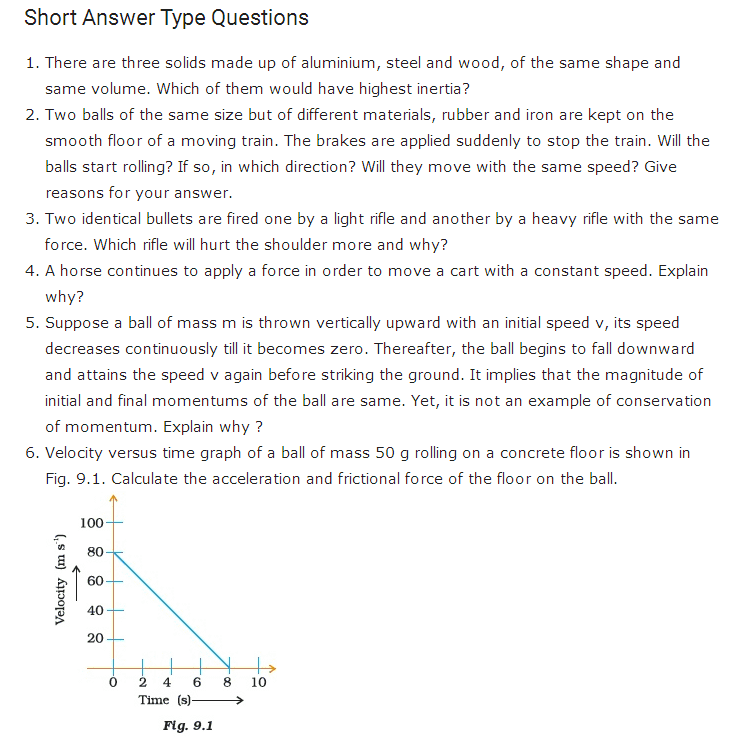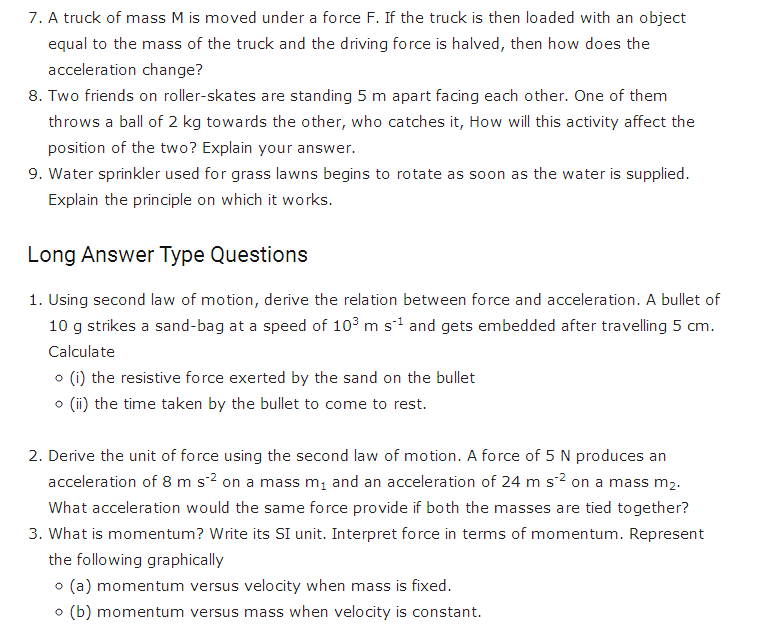# Important Questions for Class 9 Science Chapter 9 - Force and Laws of Motion

In CBSE Class 9 Science Chapter 9 – Force and Laws of Motion, students will study topics related to the laws of motion, inertia and mass, momentum, etc. Subject-matter experts have prepared these important questions to help students scoring well in exam. Solving these important questions of CBSE Class 9 Science after they complete the syllabus will help them to evaluate their preparation. We at BYJU’S have provided these CBSE Class 9 Science important questions in Chapter 9 so that students can refer to it while revising the concepts of Force and Laws of Motion.

Below we have provided the PDF link to download the Important Questions for CBSE Class 9 Science Chapter 9 – Force and Laws of Motion.

## Important Questions for Class 9 Science Chapter 9 – Force and Laws of Motion PDF### CBSE Class 9 Science Chapter 9 MCQs

1. An object of mass 2 kg is sliding with a constant velocity of 4 m/s on a friction less horizontal table. Calculate the force required to keep the object moving with the same velocity.

(a) 32 N

(b) 0 N

(c) 2 N

(d) 8 N

2. _______   is the unit of measuring the momentum of a moving body.

(a) m/s

(b) kg.m/s

(c) kg.m/s2

(d) N m2/kg2

3. What does the inertia of a moving object depend on?

(a) Speed of the object

(b) Momentum of the object

(c) Shape of the object

(d) Mass of the object